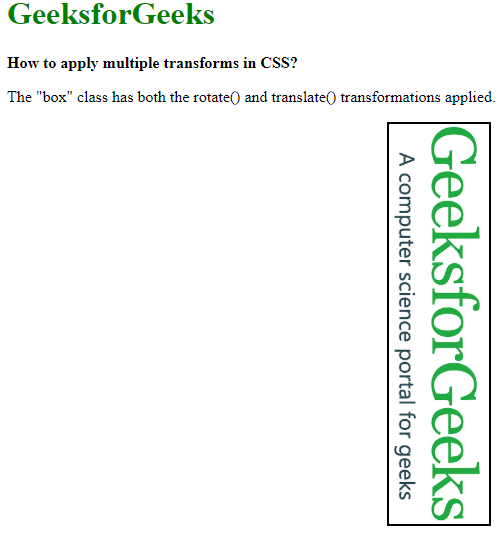# How to apply multiple transform property to an element using CSS ?

There is a variety of procedures to apply multiple transform property in an element. In this article we will discuss the easiest and popular ones. In the 1st method we will be combining the transform property itself. In the 2nd method, we will use the nested classes to apply other transforms.

Method 1: In this method, we will combine the transform property. The transform property can be given multiple values that are applied one after another. It applies the rightmost value and then the ones on the left, which means that the value which is last in the list would be applied first. This is important as changing the order of the values would change the overall result of the property.

• Example: In this example we will apply both the transform property on the loaded image, one will rotate the image and another one will shift the image.

 ` ` `<``html``> ` ` `  `<``head``> ` `    ``<``style``> ` `        ``body { ` `            ``margin: 20px; ` `        ``} ` `         `  `        ``.box { ` `            ``background: url( ` `"https://media.geeksforgeeks.org/wp-content/uploads/20191227120549/gfg77.png") ` `              ``no-repeat; ` `            ``background-size: cover; ` `            ``height: 100px; ` `            ``width: 400px; ` `            ``border: 2px solid black; ` `           `  `            ``/* The transformations are  ` `               ``applied from right to left */ ` `            ``transform: rotate(90deg)  ` `                       ``translate(150px, -230px); ` `        ``} ` `         `  `        ``h1 { ` `            ``color: green; ` `        ``} ` `    `` ` ` ` ` `  `<``body``> ` `    ``<``h1``> ` `        ``GeeksforGeeks ` `    `` ` `    ``<``b``> ` `        ``How to apply multiple transforms in CSS? ` `    `` ` `    ``<``p``> ` `        ``The "box" class has both the rotate() and ` `        ``translate() transformations applied. ` `    `` ` `    ``<``div` `class``=``"box"``> ` ` ` ` `  ` `

• Output:Method 2: Here we are using nested classes to apply other transforms. This method works by nesting one element with a certain transform with another one with another transform. This can be repeated with multiple nesting of elements to apply multiple transforms. The topmost element, that is the parent of the nested elements would be applied first and then subsequently each of the children’s transforms would be applied next.

• Example: In this example, we will apply both the transform property on the loaded image one will rotate the image and another one will shift the image.

 ` ` `<``html``> ` ` `  `<``head``> ` `    ``<``style``> ` `        ``.outer-transform { ` `           `  `            ``/* This transformation ` `               ``will be applied first */ ` `            ``transform: translate(-150px, 150px); ` `        ``} ` `         `  `        ``.inner-transform { ` `            ``background: url( ` `"https://media.geeksforgeeks.org/wp-content/uploads/20191227120549/gfg77.png")  ` `              ``no-repeat; ` `            ``background-size: cover; ` `            ``height: 100px; ` `            ``width: 400px; ` `            ``border: 2px solid black; ` `           `  `            ``/* This transformation ` `               ``would be applied next */ ` `            ``transform: rotate(-90deg); ` `        ``} ` `    `` ` ` ` ` `  `<``body``> ` `    ``<``h1` `style``=``"color: green;"``> ` `        ``GeeksforGeeks ` `    `` ` `    ``<``b``> ` `        ``How to apply multiple transforms in CSS? ` `    ``     ` `    ``<``p``> ` `        ``The inner element has the rotate() transformation and ` `        ``<``br``> the outer element has the translate() transformation ` `         ``applied. ` `    `` ` `    ``<``div` `class``=``"outer-transform"``> ` `        ``<``div` `class``=``"inner-transform"``> ` `    `` ` ` ` ` `  ` `

• Output:My Personal Notes arrow_drop_upCheck out this Author's contributed articles.

If you like GeeksforGeeks and would like to contribute, you can also write an article using contribute.geeksforgeeks.org or mail your article to contribute@geeksforgeeks.org. See your article appearing on the GeeksforGeeks main page and help other Geeks.

Please Improve this article if you find anything incorrect by clicking on the "Improve Article" button below.